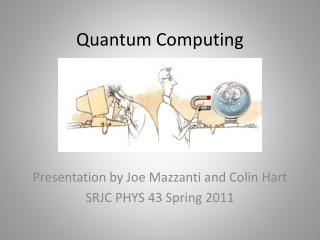DownloadDownload PresentationQuantum Computing

# Quantum Computing

Télécharger la présentation## Quantum Computing

- - - - - - - - - - - - - - - - - - - - - - - - - - - E N D - - - - - - - - - - - - - - - - - - - - - - - - - - -
##### Presentation Transcript

1. Quantum Computing Presentation by Joe Mazzanti and Colin Hart SRJC PHYS 43 Spring 2011

2. A little background… • A computer is a programmable machine designed to sequentially and automatically carry out a sequence of arithmetic or logical operations. • Conventionally a computer consists of some form of memory for data storage, at least one element that carries out arithmetic and logic operations, and a sequencing and control element that can change the order of operations based on the information that is stored. • A computer's processing unit executes series of instructions that make it read, manipulate and then store data. • Historically, computers evolved from mechanical computers and eventually from vacuum tubes to transistors. Modern computers are usually made out of transistors made of photolithographed semiconductors.

3. What is Quantum Computing? • Definition - Use of quantum phenomenon to perform computational operations • Operations are done at an atomic level

4. Physics in Quantum Computing • Properties employed: • Quantum superposition • Quantum entanglement • Uncertainty principal • Wave interference

5. Quantum based vs. Transistor based • Bit = 0 or 1. Equivalent to switching a light switch on and off. • Qubit = 0 and/or 1 . • This superposition of states is what makes quantum computing fundamentally different.

6. The Bloch Sphere • The Bloch Sphere is a representation of a qubit, the fundamental building block of quantum computers.

7. Y=2n • By performing the single operation on the qubit, we have performed the operation on two different values. Likewise, a two-qubit system would perform the operation on 4 values, and a three-qubit system on eight. • Increasing the number of qubits therefore exponentially increases the 'quantum parallelism' we can obtain with the system

8. Heroes of Quantum Computing • Richard Feynman In 1980, Richard Feynman, among others, begins to investigate the generalization of conventional information science concepts to quantum physical processes, considering the representation of binary numbers in relation to the quantum states of two-state quantum systems: in other words, simulating quantum systems not with conventional computers but with other quantum systems constructed for this purpose.

9. Before we continue… • A logic gate is an idealized or physical device implementing a Boolean function, that is, it performs a logical operation on one or more logic inputs and produces a single logic output.

10. Heroes of Quantum Computing • David Deutsch In 1985, David Deutsch, publishes a theoretical paper describing a universal quantum computer, proving that if two-state system could be made to evolve by means of a set of simple operations, any such evolution could be produced, and made to simulate any physical system; these operations come to be called quantum 'gates', as they function similarly to binary logic gates in classical computers.

11. Heroes of Quantum Computing • Peter Shor In 1994, proposes a method using entanglement of qubits and superposition to find the prime factors of an integer, a rather valuable process as many encryption systems exploit the difficulty in finding factors of large numbers.  In principle, his algorithm would far surpass the efficiency of any known computer when executed on a quantum computer; Shor’s discovery proves quite instrumental in provoking a storm of research both by physicists and computer scientists.

12. Developing Algorithms

13. Where are we now? • There is active research to make computers out of many promising new types of technology, such as:  • Optical computing • DNA computers • neural computers • quantum computers • Some of these can easily tackle problems that modern computers cannot. • A 2-bit quantum computer has been made from a thimble of chloroform; its input consists of radio frequency pulses into the liquid containing, in essence, the compiled program to be executed.

14. Applications of Quantum Computing • Parallel computations • Increase efficiency for iterative repetitive tasks: • Exponential growth per qubit • Factoring • Cryptography • Cracking RSA encryption • Sorting • Huge database management • Identifying singularities • Molecules, knot theory, etc. • Game tree evaluation • Quantum simulation

15. Limitations of Quantum Computing • The Moore’s Law nano-scale conundrum: quantum tunneling

16. The (De)Coherence Problem • 1995 - The National Institute of Standards and Technology and the California Institute of Technology jointly contemplate the problem of shielding a quantum system from environmental influences  and perform experiments with magnetic fields, which allow particles (ions) to be trapped and cooled to a quantum state.  This method, however, allows only devices of a few bits to be created, ones which lose coherence rapidly.

17. Decoherence • Decoherence can be viewed as the loss of information from a system into the environment (often modeled as a heat bath). It is thus acknowledged that no system is, in reality, perfectly isolated—but rather every system is loosely coupled with the energetic state of its surroundings. Viewed in isolation, the system's dynamics are non-unitary (although the combined system plus environment evolves in a unitary fashion). Thus the dynamics of the system alone, treated in isolation from the environment, are irreversible. As with any coupling, entanglements are generated between the system and environment, which have the effect of sharing quantum information with—or transferring it to—the surroundings.

18. Limitations of Quantum Computing • Reliance on coherence • Devolving into classical states • Avoiding this relies on small components • Alternatively, state can be preserved using very cold temperatures of operation • Very hard materials (Diamond)

19. Current Events • Current method for creating qubits • Ion Traps • Optical Traps • Quantum dots • Semiconductor impurities • Superconducting circuits • D-Wave 16 Qubit Quantum Computer • Demonstrated in 2010 • Solved a Sudoku Puzzle • Qlisp- quantum programming language